# C Program to Print a Series of N Terms

Here we will learn about how to print some series up to the given term (by the user at run-time). Here is a list of some programs that will ask the user to enter the value of N (where N is the limit or term up to which the series will be printed) to print the series up to that term.

## C Print 1, 2, 3, 4, and so on up to N terms

Here is the program that will print the series 1, 2, 3, 4,... up to N (as given by the user at run-time):

```#include<stdio.h>
#include<conio.h>
int main()
{
int N, i;
printf("Enter the value of N (limit): ");
scanf("%d", &N);
printf("\n");
for(i=1; i<=N; i++)
{
if(i==N)
printf("%d", i);
else
printf("%d,", i);
}
getch();
return 0;
}```

As the above program was written in the Code::Blocks IDE, here is the sample run after a successful build and run. This is the first snapshot of the sample run: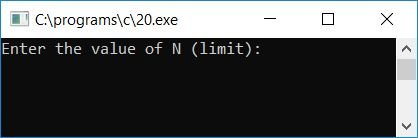Now provide the limit, say 25, to print the series up to the given term as shown here in the second snapshot of the sample run: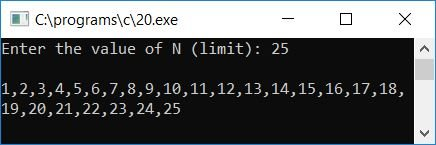Here are some of the main steps used in the above program:

• Receive the value of N to print the series up to that given term.
• Create a for loop that runs from 1 to the given number, which is N.
• Inside the loop, print the value of i one by one.

## C Print 1, 4, 9, 16,... all the way up to N Term

Here is another program that will print the series 1, 4, 9, 16,... up to N. The value of N will be provided by the user at run-time.

```#include<stdio.h>
#include<conio.h>
int main()
{
int N, i, inc=1, val=1, temp;
printf("Enter the value of N (limit): ");
scanf("%d", &N);
printf("\n");
for(i=1; i<=N; i++)
{
if(i>1)
{
inc = inc+2;
temp = inc;
val = val+temp;
}
if(i==N)
printf("%d", val);
else
printf("%d, ", val);
}
getch();
return 0;
}```

Here is the final snapshot of the sample run of the above program: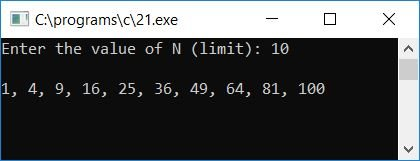Here are some of the main steps used in the above program:

• Receive the value of N, say 10, as input to print the series up to that given term (10 times here).
• Create a for loop that runs from 1 to N.
• Inside the for loop, check whether the loop variable i is greater than 1 or not.
• If it is, then increment a variable, say inc, by 2 each time and initialize it to the variable, say temp. And finally, add it to another variable, say val, and initialize it to the variable val, which will be the current value to be printed as output.
• Never forget to initialize 1 to both the variables, say, inc and val, at the start of the program.
• Therefore, at the first run of the for loop, i holds 1, which is not greater than 1. Therefore, the program flow does not go inside the if block, and the value of i will be printed directly.
• At the second run, i holds 2, which is greater than 1, therefore the program flow goes inside the if block, and inc+2 or 1+2 or 3 will be initialized to inc, and inc or 3 will be initialized to temp, and val+temp or 1+3 or 4 will be initialized to val. Finally, print the value of val, which is 4.
• At the third run, i holds 3, which is again greater than 1, therefore inside the if block, inc+2 or 3+2 or 5 will be initialized to inc, inc or 5 will be initialized to temp, and val+temp or 4+5 or 9 will be initialized to val. Finally print the value of val and continue to check for the next value of i.
• In this way, the series gets printed as output.

## Print the series: 0, 2, 6, 12,... N in C

Let's create another program that prints another series that is 0, 2, 6, 12,... up to N terms:

```#include<stdio.h>
#include<conio.h>
int main()
{
int N, temp, inc=0, val=0, i;
printf("Enter the value of N (limit): ");
scanf("%d", &N);
for(i=1; i<=N; i++)
{
if(i>1)
{
inc = inc + 2;
temp = inc;
val = val + temp;
}
if(i==N)
printf("%d", val);
else
printf("%d, ", val);
}
getch();
return 0;
}```

Here is a sample run of the above program: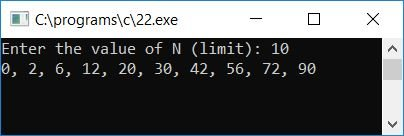Use the same steps to create the above program, except that instead of 1, initialize 0 to both the variables val and inc (in this program).

## C Print 1, 8, 27, 64,... all the way up to N Term

Let's create another program to print another series that is 1, 8, 27, 64,... up to N:

```#include<stdio.h>
#include<conio.h>
int main()
{
int N, i, val;
printf("Enter the value of N (limit): ");
scanf("%d", &N);
for(i=1; i<=N; i++)
{
val = i*i*i;
if(i==N)
printf("%d", val);
else
printf("%d, ", val);
}
getch();
return 0;
}```

This is the final snapshot of the sample run of the above program: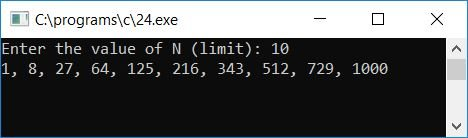## C Print 1, 2, 4, 7,... all the way up to N Term

Let's create one more program to print one more series that is 1, 2, 4, 7, 11, 16,... up to N in C.

```#include<stdio.h>
#include<conio.h>
int main()
{
int N, i, val=1, temp, inc=1;
printf("Enter the value of N (limit): ");
scanf("%d", &N);
for(i=1; i<=N; i++)
{
if(i>1)
{
val = val + inc;
inc++;
}
if(i==N)
printf("%d", val);
else
printf("%d, ", val);
}
getch();
return 0;
}```

This is a snapshot of the sample run: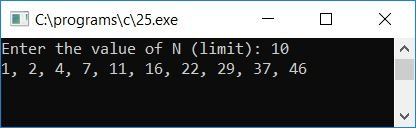C Quiz

« Previous Program Next Program »